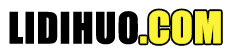# 搜索

 技术与说明 线性搜索 线性搜索搜索所有项目，其最差执行时间为n，其中n是项目数。 二分搜索 二分搜索要求项目按排序顺序，但其最差执行时间是恒定的，并且比线性搜索快得多。 插值搜索 插值搜索要求项目按排序顺序，但其最差执行时间是 O(n)，其中 n 是items，它比线性搜索快得多。

## 线性搜索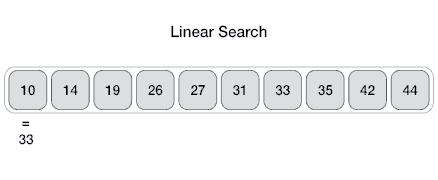### 算法

```Linear Search ( Array A, Value x)
Step 1: Set i to 1
Step 2: if i > n then go to step 7
Step 3: if A[i] = x then go to step 6
Step 4: Set i to i + 1
Step 5: Go to Step 2
Step 6: Print Element x Found at index i and go to step 8
Step 8: Exit
```

### 伪代码

```procedure linear_search (list, value)
for each item in the list
if match item == value
return the item's location
end if
end for
end procedure
```

## 二分搜索

### 二分搜索如何工作？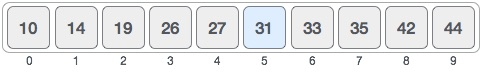```mid = low + (high-low) / 2
```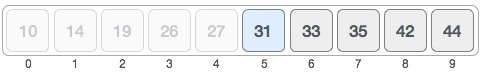```low = mid + 1
mid = low + (high-low) / 2
```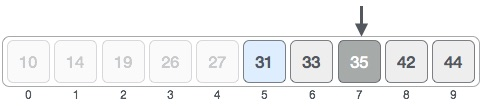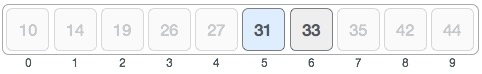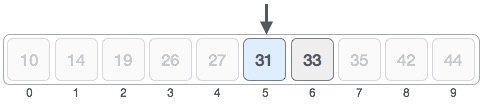### 伪代码

```Procedure binary_search
A ← sorted array
n ← size of array
x ← value to be searched
Set lowerBound = 1
Set upperBound = n
if upperBound < lowerBound
EXIT: x does not exists.

set midPoint = lowerBound + ( upperBound-lowerBound ) / 2

if A[midPoint] < x
set lowerBound = midPoint + 1

if A[midPoint] > x
set upperBound = midPoint -1
if A[midPoint] = x
EXIT: x found at location midPoint
end while

end procedure
```

## 插值搜索

### 二分查找中的定位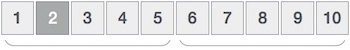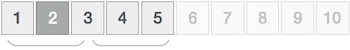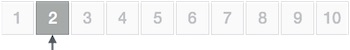### 插值搜索中的位置探测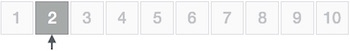```mid = Lo + ((Hi-Lo) / (A[Hi]-A[Lo])) * (X-A[Lo])
where −
A    = list
Lo   = Lowest index of the list
Hi   = Highest index of the list
A[n] = Value stored at index n in the list
```

### 算法

```Step 1 − Start searching data from middle of the list.
Step 2 − if it is a match, return the index of the item, and exit.
Step 3 − if it is not a match, probe position.
Step 4 − Divide the list using probing formula and find the new midle.
Step 5 − if data is greater than middle, search in higher sub-list.
Step 6 − if data is smaller than middle, search in lower sub-list.
Step 7 − Repeat until match.
```

### 伪代码

```A → Array list
N → Size of A
X → Target Value
Procedure Interpolation_Search()
Set Lo  →  0
Set Mid →-1
Set Hi  →  N-1
while X does not match

if Lo equals to Hi OR A[Lo] equals to A[Hi]
end if

Set Mid = Lo + ((Hi-Lo) / (A[Hi]-A[Lo])) * (X-A[Lo])
if A[Mid] = X
EXIT: Success, Target found at Mid
else
if A[Mid] < X
Set Lo to Mid+1
else if A[Mid] > X
Set Hi to Mid-1
end if
end if
End While
End Procedure
```